c语言结构：
1. 顺序结构
2. 选择结构
3. 循环结构

## 分支语句与循环语句：

c语言中由一个分号隔开的，就是一个语句

## if语句结构：

1.if（表达式）

2.if（表达式）

else

3.多分支
.if（表达式1）

else if（表达式2）

else

``````#define _CRT_SECURE_NO_WARNINGS 1
#include<stdio.h>
int main()
{

int age;
printf("please input your age\n");
scanf("%d", &age);
if (age < 18)
printf("未成年\n");
else if (age >= 18 && age < 28)//&&为“和”
printf("青年\n");
else if (age >= 28 && age < 50)
printf("壮年\n");
else if (age >= 50 && age < 90)
printf("老年\n");
else
printf("老不死\n");
return 0;
}
``````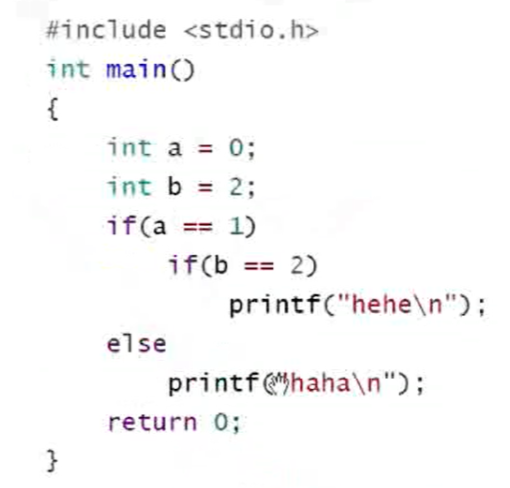## switch语句

(只能定义整型）

case决定入口，break决定出口

``````#define _CRT_SECURE_NO_WARNINGS 1
#include<stdio.h>
int main()
{

int day;
scanf("%d", &day);
switch (day)
{

case 1:
printf("星期1\n");
break;
case 2:
printf("星期2\n");
break;
case 3:
printf("星期3\n");
break;
case 4:
printf("星期4\n");
break;
case 5:
printf("星期5\n");
break;
case 6:
printf("星期6\n");
break;
case 7:
printf("星期日\n");
break;

}
return 0;
}
``````

case 2:
case 3:
case 4:
case 5:
printf(“工作日\n”);
break;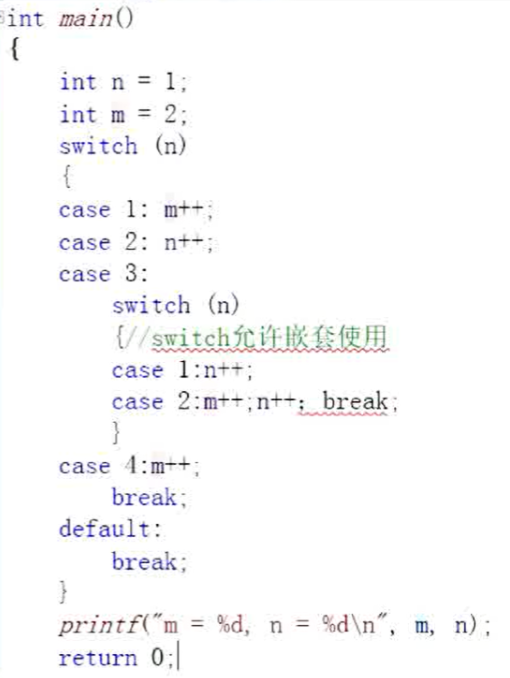m=5 n=3

## while循环

``````while（i<=10）
{

if(i==5) ;
break;
printf("%d",1):
i++;
}
``````

getchar-输入一个字符并返回（待输入）
putchar-输出

``````int ch =0;
while ((ch=getchar())!=EOF)
{

putchar(ch);
}
``````

（EOF–end of file文件结束标志）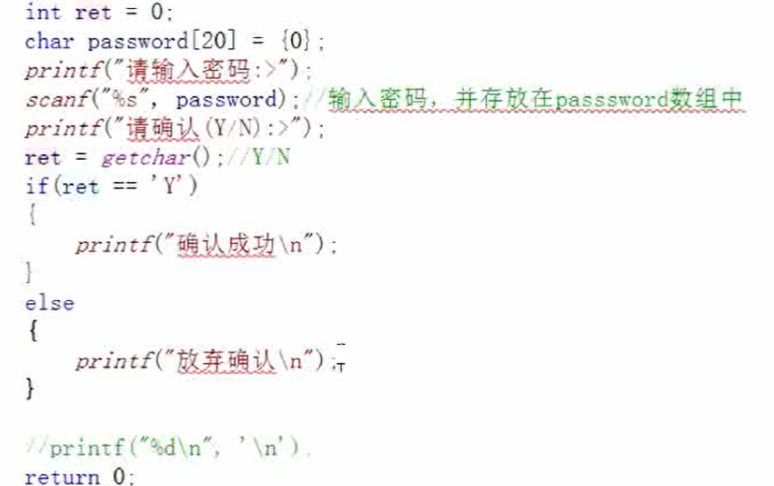getchat和scanf为输入函数

getchar得到缓冲区剩的回车（\n）去到else输出失败

``````int ch
while（（ch=getchar（））！=‘\n’）//一直拿到回车
{

..........(上述题中的代码）
}
``````

（这道题对初学者来讲较有难度，这里放出来是为了让大家体会计算机思维，如果仔细看完解释还是不懂可以在评论区中提出来你的问题）

## for循环

（用的最多）

for（表达式1；表达式2；表达式3）

*

*

*

eg：用for循环打印1-10

``````#include<stdio.h>
int main()
{

int i = 0;
for (i = 1; i <= 10; i++)
{

printf("%d", i);
}
return 0;
}
``````

for循环示意图：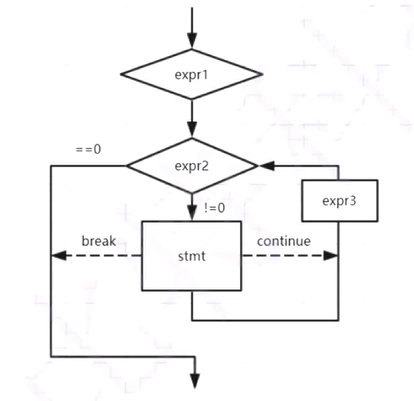2.建议for循环控制变量取值采用“前闭后开区间”写法（前等后不等）----更有意义
3.for循环的判断条件部分若被省略，条件恒为真，循环不会停！(建议不要随便省略）初始化省略后默认从0开始

## do…while循环

``````int i=1
do
{

printf("%d",1);
i++;
}

while(i<=10);//适用范围

retrun 0;
``````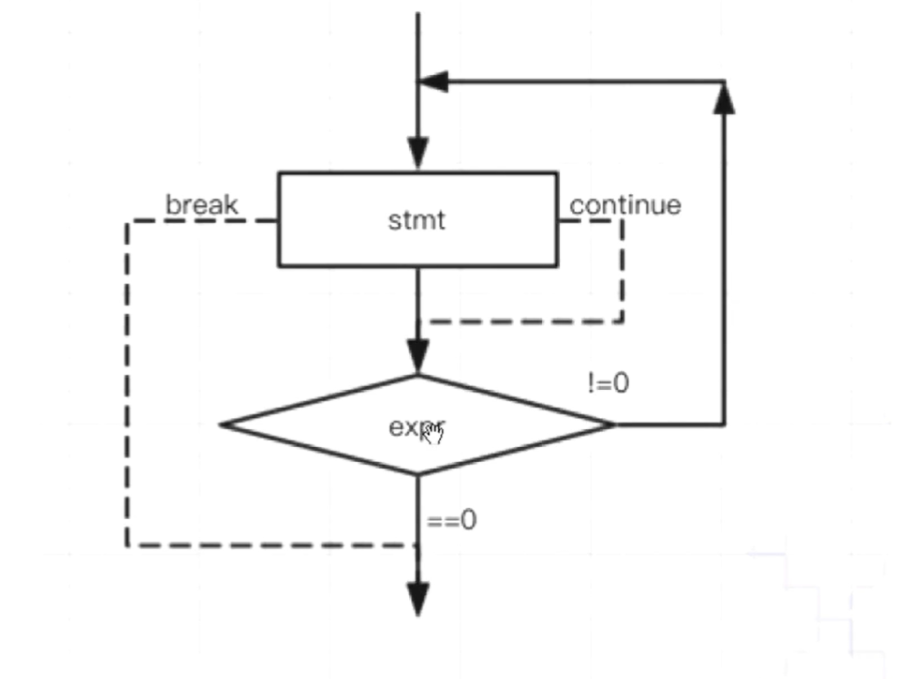## 练习

1.计算n的阶乘（不考虑溢出）
2.在有序数组中查找某个具体的数字n，编写int binsearch（int x,int v[],int n）;功能：在v<=v<=v<=…<=v[n-1]的数组中查找x。
3.编写代码演示多个字符从两边汇聚到中间
4.模拟用户登录，输入密码，最多输入三次

## 答案

1.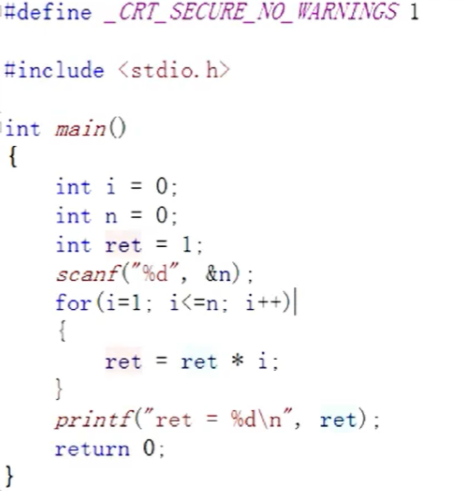• 简单版：
``````#include<stdio.h>
int main()
{

int arr[] = {

1,2,3,4,5,6,7,8,9,10 };
int k = 7;
//写一个代码，在arr数组（有序的）中找到7
int i = 0;
int sz = sizeof(arr) / sizeof(arr);
for (i = 0; i < sz; i++)
{

if (k == arr[i])
{

printf("找到了，下标是；%d\n", i);
break;
}
}
if (i == sz)
printf("找不到\n");
return 0;
}
``````
• 折半（二分）查找算法

``````#include<stdio.h>
int main()
{

int arr[] = {

1,2,3,4,5,6,7,8,9,10 };
int k = 7;
int sz = sizeof(arr) / sizeof(arr);//计算元素个数
int left = 0;//定义左下标
int right = sz - 1;//定义右下标

while (left<=right)
{

int mid = (left + right) / 2;
if (arr[mid] > k)
{

right = mid - 1;
}
else if (arr[mid] < k)
{

left = mid + 1;
}
else
{

printf("找到了，下标是：%d\n", mid);
break;
}
}
if(left>right)
{

printf("找不到\n");
}
return 0;
}
``````

3，

``````#include<stdio.h>
#include<string.h>
#include<CoreWindow.h>//sleep 的声明
#include<stdlib.h>//system的声明
int main()
{

char arr1[] = "welcome to china!!!!!";
char arr2[] = "#####################";
int left = 0;
//int right = sizeof(arr1) / sizeof(arr1) - 2;//算上\0所以减2
int right = strlen(arr1) - 1;//strlen为计算数组中元素个数的函数，更简便
while (left <= right)
{

arr2[left] = arr1[left];
arr2[right] = arr1[right];
printf("%s\n", arr2);
Sleep(1000);//间隔1000毫秒
system("cls");//执行系统命令的一个函数-cls-清空屏幕"cls"
left++;
right--;
}
return 0;
}
``````
``````#define _CRT_SECURE_NO_WARNINGS 1
#include<stdio.h>
#include<string.h>//strcmp的库函数
int main()
{

int i = 0;
char password = {

0 };
for (i = 0; i < 3; i++)
{

printf("请输入你的密码");
scanf("%s", password);//输入字符串
if (strcmp(password , "123456")==0)//等号不能用来判断两个字符串是否相等，应用“strcmp”函数来判断
{

printf("登陆成功\n");
break;
}
else
{

printf("密码错误\n");
}
}
if (i == 3)
printf("三次密码均错误，请退出");
return 0;
}
``````